# Section 8.2. Formula Shortcuts

### 8.2. Formula Shortcuts

So far, you've learned how to build a formula by entering it manually. That's a good way to start out because it forces you to understand the basics of formula writing. But writing formulas by hand is a drag; plus, it's easy to type in the wrong cell address. For example, if you type A2 instead of A3, you can end up with incorrect data, and you won't necessarily notice your mistake.

As you become more comfortable with formulas, you'll find that Excel gives you a few toolslike point-and-click formula creation and the Insert Function buttonto speed up your formula writing and reduce your mistakes. You'll learn about these features in the following sections.

Note: In previous versions of Excel, the Insert Function dialog box was almost exactly the same, except it was known as the Function wizard.

#### 8.2.1. Point-and-Click Formula Creation

Instead of entering a formula by typing it out letter-by-letter, Excel lets you create formulas by clicking the cells you want to use. For example, consider this simple formula that totals the numbers in two cells:

` =A1+A2 `

To build this formula by clicking, just follow these steps:

1. Move to the cell where you want to enter the formula .

This cell's where the result of your formula's calculation will appear. While you can pick any cell on the worksheet, A3 works nicely because it's directly below the two cells you're adding.

2. Press the equal sign (=) key .

The equal sign tells Excel you're going to enter a formula.

3. Move to the first cell you want to use in your formula (in this case, A1) .

You can move to this first cell by pressing the up arrow key twice, or by clicking it with the mouse. You'll notice that moving to another cell doesn't cancel your edit, as it would normally, because Excel recognizes that you're building a formula. When you move to the new cell, the cell reference appears automatically in the formula (which Excel displays in cell A3, as well as in the formula bar just above your worksheet). If you move to another cell, Excel changes the cell reference accordingly .

4. Press the + key .

5. Finish the formula by moving to cell A2 and pressing Enter .

Again, you can move to A2 either by pressing the up arrow key or by clicking the cell directly. Remember, you can't just finish the formula by moving somewhere else; you need to press Enter to tell Excel you're finished writing the formula. Another way to complete your edit is to click the checkmark that appears on the formula bar, to the left of the current formula. Even experienced Excel fans get frustrated with this step. If you click another cell before you press Enter, then you won't move to the cellinstead, Excel inserts the cell into your formula.

Tip: You can use this technique with any formula. Just type in the operators, function names , and so on, and use the mouse to select the cell references. If you need to select a range of cells, then just drag your mouse until the whole group of cells is highlighted. You can practice this technique with the SUM( ) function. Start by typing =SUM( into the cell, and then selecting the range of cells you want to add. Finish by adding a final closing parenthesis and pressing Enter.

#### 8.2.2. Point-and-Click Formula Editing

You can use a similar approach to edit formulas, although it's slightly trickier.

1. Move to the cell that contains the formula you want to edit, and put it in edit mode by double-clicking it or pressing F2 .

Excel highlights all the cells that this formula uses with a colored outline. Excel's even clever enough to use a helpful color-coding system. Each cell reference uses the same color as the outline surrounding the cell it's referring to. This can help you pick out where each reference is.

2. Click the outline of the cell you want to change. (Your pointer changes from a fat plus sign to a four-headed arrow when you're over the outline.) With the mouse button still held down, drag this outline over to the new cell (or cells) you want to use .

Excel updates the formula automatically. You can also expand and shrink cell range references. To do so, put the formula-holding cell into edit mode, and then click any corner of the border that surrounds the range you want to change. Next , drag the border to change the size of the range. If you want to move the range, then click any part of the range border and drag the outline in the same way as you would with a cell reference.

3. Press Enter or click the formula bar checkmark to accept your changes .

That's it.

#### 8.2.3. The Formulas Tab

The ribbon is stocked with a few buttons that make formula writing easier. To take a look, click the Formulas tab.

 FREQUENTLY ASKED QUESTION Showing and Printing Formulas How in the world do I print out formulas that appear in my cells ? When you print a worksheet, Excel prints the calculated value in each cell rather than any formula that happens to be inside a cell. Usually, that's what you want to have happen. But in some cases, rather than a printout of the formula's results, you want a record of the calculations used to generate the results. Excel gives you a view setting so you can get this record. Just choose Formulas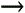Formula AuditingShow Formulas. Now, Excel displays the formulas contents instead of its resultsbut on the current worksheet only. Excel also widens the columns so they can show more information (as formulas tend to be longer than their results). Repeat this process, and then uncheck the setting to return to normal life.

The most important part of the Formulas tab is the Function Library section at the left. It includes the indispensable Insert Function button, which you'll take for a spin in the next section. It also includes many more buttons that arrange Excel's vast catalog of functions into related categories for easier access. Figure 8-7 shows how it works.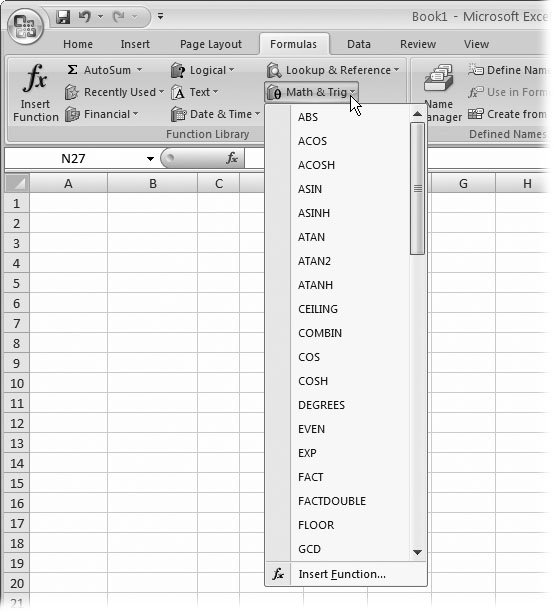Figure 8-7. Each button in the Function Library section (other than Insert Function) pops up a mini menu of function choices. Choose one, and Excel inserts that function into the current formula. You can use this technique to find functions that you've used recently, or to browse the main function categories. This example shows some of the functions in the Math & Trig section, which you'll explore in the next chapter.

The Function Library divides its functions into the following categories:

• AutoSum has a few shortcuts that let you quickly add, average, or otherwise deal with a list of numbers. You'll see how they work in Section 9.2.2.

• Recently Used has exactly what you'd expectfunctions that you've recently chosen from the Function Library. If you're just starting out with functions, then you see that Excel fills the Recently Used list with a small set of commonly used functions, like SUM( ).

• Financial functions let you track your car loan payments and calculate how many more years until you can retire rich. You'll tackle them in Chapter 10.

• Logical functions let you create conditional logic for even smarter spreadsheets that make calculation decisions. You already had a quick introduction to condition logic earlier in this chapter, but you'll get more details in Chapter 13.

• Text functions manipulate words, sentences, and other non-numeric information. Chapter 11 has the scoop.

• Date & Time functions perform calendar math, and can help you sort out ages, due dates, and more. You'll try them out in Chapter 11.

• Lookup & Reference functions perform the slightly mind-bending feat of searching for information in other cells. You'll see why they're so useful in Chapter 12.

• Math & Trig functions are the mathematic basics, including sums, rounding, and all the other high-school trigonometry you're trying to forget. Chapter 9 explores this section.

• More Functions groups together some heavy-duty Excel functions that are intended for specialized purposes. This category includes high- powered statistical and engineering functions. It also includes cube functions, which are designed for working highly technical OLAP ( online analytical processing ) databases, and information functions, which get "information" about the other cells in your worksheet. You won't see all of these functions in this book, because some are reserved for true specialists, but you'll dip your toe into a few statistical functions in Chapter 9, and you'll explore information functions in Chapter 12.

The Function Library section isn't the only part of the Formulas tab that you'll use. To the right are buttons for using named cells, tracking down errors, and changing calculation settings (three techniques you'll pick up in Chapter 13).

#### 8.2.4. Using the Insert Function Button

Excel provides more than 300 built-in functions. In order to use a function, however, you need to type its name in exactly . That means that every time you want to employ a function, you'll need to refer to this book, call on your own incredible powers of recollection, or click over to the convenient Insert Function button.

To use the Insert Function feature, choose FormulasFunction LibraryInsert Function. However, formula pros skip straight to the action by clicking the fx button that appears just to the left of the formula bar. (Or, they press the Shift+F3 shortcut key.)

No matter which approach you use, Excel displays the Insert Function dialog box (shown in Figure 8-8), which offers three ways to search for and insert any of Excel's functions.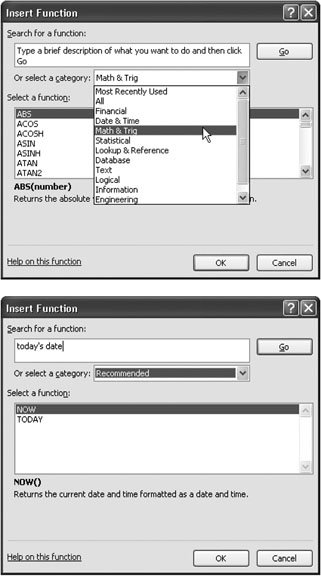Figure 8-8. Top: The Insert Function dialog box lets you quickly find the function you need. You can choose a category that seems likely to have the functions you're interested in. Bottom: You can also try to search by entering keywords in the "Search for a function" box. Either way, when you click one of the functions in the list, Excel presents you with a description of the function at the bottom of the window.

• If you're looking for a function, the easiest way to find one is to choose a category from the "Or select a category" drop-down list. For example, when you select the Math & Trig category, you see a list of functions with names like SIN( ) and COS( ), which perform basic trigonometric calculations.

• If you choose the Most Recently Used category, you'll see a list of functions you've recently picked from the ribbon or the Insert Function dialog box.

• If you're really ambitious, you can type a couple of keywords into the "Search for a function" text box. Next, click Go to perform the search. Excel gives you a list of functions that match your keywords.

When you spot a function that looks promising , click it once to highlight its name. Excel then displays a brief description of the function at the bottom of the window. For more information, you can click the "Help on this function" link in the bottom-left corner of the window. To build a formula using this function, click OK.

Excel then inserts the function into the currently active cell, followed by a set of parentheses. Next, it closes the Insert Function dialog box and opens the Function Arguments dialog box (Figure 8-9).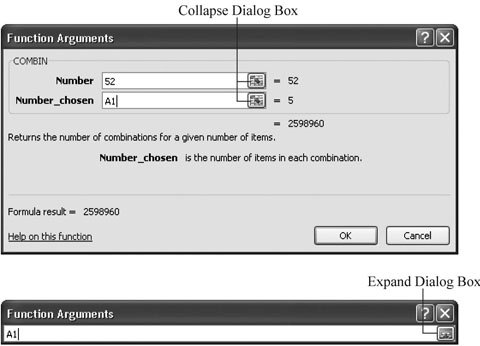Figure 8-9. Top: Here, the COMBIN( ) function has just been inserted via the Insert Function dialog box. Because the COMBIN( ) function requires two arguments (Number and Number_chosen), the Function Arguments dialog box shows two text boxes. The first argument uses a literal value (52), while the second argument uses a cell reference (A1). (You can use literal values or a cell reference for either argumentit's up to you.) As you enter the arguments, Excel updates the formula in the worksheet's active cell, and displays the result the calculation at the bottom of the Function Arguments dialog box. Bottom: If you need more room to see the worksheet and select cells, you can click the Collapse Dialog Box icon to reduce the window to a single text box. Clicking the Expand Dialog Box icon restores the window to its normal size.

Note: Depending on the function you're using, Excel may make a (somewhat wild) guess about which arguments you want to supply. For example, if you use the Insert Function window to add a SUM( ) function, then you'll see that Excel picks a nearby range of cells. If this isn't what you want, just replace the range with the correct values.

Now you can finish creating your formula by using the Function Arguments dialog box, which includes a text box for every argument in the function. It also includes a help link for detailed information about the function, as shown in Figure 8-10.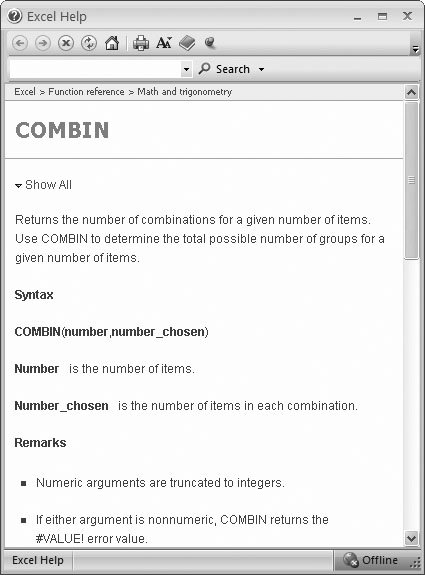Figure 8-10. Both the Insert Function and Function Arguments dialog boxes make it easy to get detailed reference information about any function by clicking the "Help on this function" link at the bottom left of the window. The help page shown here shows the reference for the trigonometric SIN( ) function, which calculates the sine of an angle. Excel's help page includes a brief description, important notes, and a couple of sample formulas that use the function, complete with results.

1. Click the text box for the first argument .

A brief sentence describing the argument appears in the Function Arguments dialog box.

Some functions don't require any arguments. In this case, you don't see any text boxes, although you still see some basic information about the function. Skip directly to step 4.

2. Enter the value for the argument .

If you want to enter a literal value (like the number 52), type it in now. To enter a cell reference, you can type it in manually, or click the appropriate cell on the worksheet. To enter a range, drag the cursor to select a group of cells.

You may need to move the Function Arguments dialog box to the side to expose the part of the worksheet you want to click. The Collapse Dialog Box icon (located to the immediate right of each text box) is helpful since clicking it shrinks the window's size. This way, you'll have an easier time selecting cells from your worksheet. To return the window to normal, click the Expand Dialog Box icon, which is to the right of the text box.

3. Repeat step 2 for each argument in the function .

As you enter the arguments, Excel updates the formula automatically.

4. Once you've specified a value for every required argument, click OK .

Excel closes the window and returns you to your worksheet.

 POWER USERS' CLINIC Functions That Return Arrays A few exotic functions actually give you multiple results, so rather than simply generating a single value, as a function like SUM( ) would, these functions generate more than one value. To use these functions properly, you need to create a special type of formula called an array formula . (An array is a group of numbers.) You can create an array formula quite easily. Simply select all the cells that Excel will use to display the results, type in the formula, and end by pressing Ctrl+Shift+Enter. It's this final keystroke that actually creates the array formula and links the cells together. Usually, you'll see a different result appear in each cell. Technically, when you create an array formula, you have a single formula that's shared between several cells. You know that you have an array formula because the formula appears in the formula bar with curly braces { } around it whenever you move into any one of the linked cells. However, you can't edit the individual cells in the array formula. (If you try, Excel warns you that you can't change part of an array.) To make a change to an array formula, you need to select all the cells in the array, edit the formula, and then press Ctrl+Shift+Enter to recreate the array formula. Some functions that require array formulas include FREQUENCY( ) and TREND( ), which are demonstrated on pages 267 and 280, respectively; and TRANSPOSE( ), discussed in Section 12.2.9.Excel 2007[c] The Missing Manual
ISBN: 596527594
EAN: N/A
Year: 2007
Pages: 173